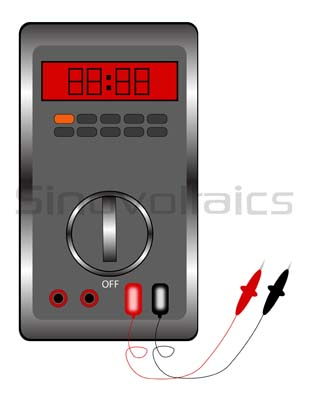# Solar Power Output Measuring

How can the solar power that a system generates be measured?

The Standard Test ConditionsMeasuring Solar Power Output of a panel depends on the solar power density falling on it, the spectral content of the solar energy, the temperature, the orientation of the panel, the area of the panel, and the load connected, and the technology used in making the panel. The power density of solar irradiance suffers an attenuation passing through the atmosphere. Also, energy at certain frequencies corresponding to water vapor resonance is attenuated more, and hence, the spectral content of sunlight also changes. For standardization of results, standard test conditions (STC) for PV panels have been fixed as 1000 watts per square meter, the solar spectral content at 1.5 AM, (Air mass, corresponding to spectrum at the earth has passed through the standard atmosphere-- as defined by IEC 60904-3), and a temperature of 25° C. Simulators are available to produce these conditions in the lab, and approximate testing can also be done outdoors under actual conditions. Corrections, if required, can be made to obtain more accurate results. To learn more: https://sinovoltaics.com/learning-center/system-design/measure-solar-energy-power/.Measure solar energy – Multimeter Power Output Power is the product of voltage and current. Both need to be measured simultaneously to provide the instant (momentary) value of power. Since the solar panel output is direct current therefore a DC power meter is required for the measurement of current. However, it is possible to calculate the power under given conditions by measuring the voltage and current output of the panel simultaneously. Then the product will give the power out. Find out more here: https://powerscout.com/site/how-to-calculate-solar-panel-output. Maximum Power It is often more important to test the panel for the maximum power it can supply under, say, STC. Maximum power occurs neither at maximum voltage nor at short circuits. In a short circuit, the voltage is zero, and hence power is zero. Maximum voltage occurs at zero current (open circuit), and hence, power is again zero. Maximum power occurs at a point close to the midpoint of the knee of the VI curve. The VI curve can be plotted by recording voltage and current simultaneously for various values of the current. The current can be varied by applying high power resistors of different values across panel output. It can also be done by applying a variable voltage (less than the open-circuit voltage) instead of the resistors.

Place comment